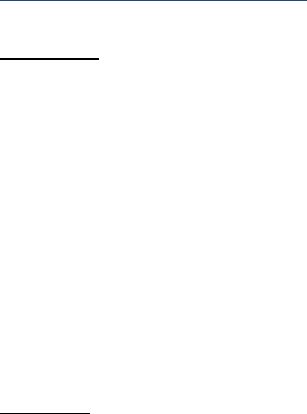# MA121 Study Guide - Midterm Guide: Distributive Property, Disjoint Sets, Symmetric Difference

182 views40 pages
School
Department
Course
Professor

Wilfrid Laurier University
MA121
Introduction to Mathematical Proofs
Fall 2017
Term Test 2
Prof: Robert John Rundle
Exam Guide
Unlock document

This preview shows pages 1-3 of the document.
Unlock all 40 pages and 3 million more documents.

Topics Covered
CHAPTER 3: SET THEORY
3.1 LOGIC VERSUS SET THEORY
3.2 SYMMETRIC DIFFERENCE
3.3 ALGEBRA OF SETS AND THE DUALITY PRINCIPLE
3.4 FINITE SETS, POWER SETS AND COUNTING PRINCIPLES
3.5 ORDERED PAIRS AND CARTESIAN PRODUCTS
3.6 FUNCTIONS AND THE PIGEONHOLE PRINCIPLE
CHAPTER 4: COMBINATORICS
4.1 PERMUTATIONS AND COMBINATIONS
4.2 BINOMIAL COEFFICIENTS
4.3 PASCAL'S TRIANGLE
4.4 THE BINOMIAL THEOREM
CHAPTER 5: NUMBER THEORY
5.2 DIVISIBILITY
5.2 DIVISION ALGORITHM
5.2 GREATEST COMMON DIVISOR
5.2 EUCLID’S ALGORITHM
5.2 FUNDAMENTAL THEOREM OF ARITHMETIC
Unlock document

This preview shows pages 1-3 of the document.
Unlock all 40 pages and 3 million more documents.CHAPTER 3: SET THEORY
3.1 LOGIC VERSUS SET THEORY
Definition: Logic is the study of deductive reasoning, the process of using mathematical
sentences to make decisions.
Let
QP,
be set and statements
qp,
as
QxPx ,
Then
qp
is the same as
QP
qp
is the same as
QP
p~
is the same as
c
P
Recall set
Theorem: Let X be an arbitrary set and let P(X) be the set of all subsets of X. P(X) is called the
power set of X. Let A, B, and C be arbitrary elements of P(X).
a. A B = B A (Commutative Law for Intersection)
A B = B A (Commutative Law for Union)
b. A (B C) = (A B) C (Associative Law for Intersection)
A (B C) = (A B) C (Associative Law for Union)
c. A B A
d. A X = A; A = A
e. A A B
f. A X = X; A =
g. A (B C) = (A B) (A C) (Distributive Law of Union with respect to Intersection)
A (B C) = (A B) (A C) (Distributive Law of Intersection with respect to Union)
h.
(A B) =
A
B
(A B) =
A
B
i. A
A = ; A
A = X
Unlock document

This preview shows pages 1-3 of the document.
Unlock all 40 pages and 3 million more documents.